# 15th LSI Design Contests・in Okinawa  Design Specification - 4-1-1

## 4-1-1. FFT circuit : N=4

### (1) Circuit design

Basic concept of 4 points FFT circuit which applies Radix-2 architecture is shown in figure 3.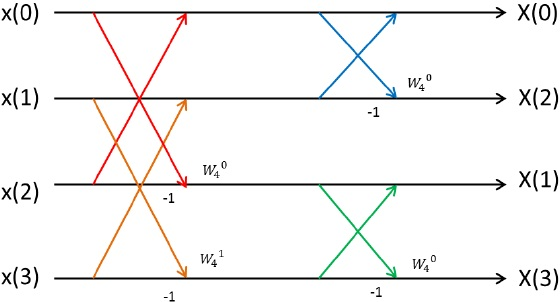Figure 3:  Basic concept of 4 points FFT circuit

In figure 3，4 point FFT circuit can implement by using 4 butterfly processing elements.

Block diagram is shown in figure 4.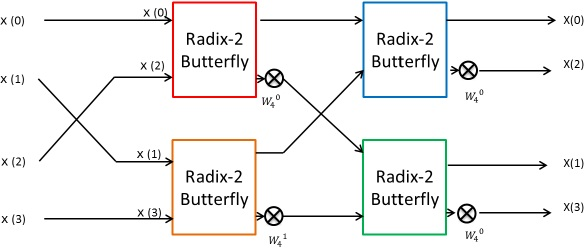Figure 4:  4 points FFT circuit block diagram

In figure 3, butterfly processing element that is shown red line make be corresponding to red block in figure 4. Another butterfly processing element is same.

This is the 4 points FFT circuit for parallel input.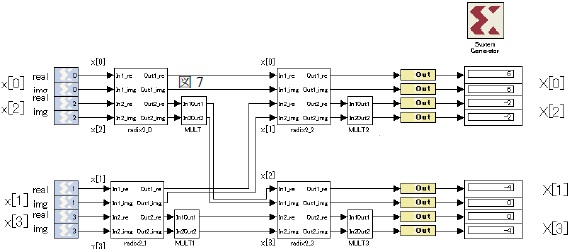Figure 5:  Design of 4 points FFT circuit for parallel input

The detail of each subsystem is shown below

In this part, we explain the Radix2_0, Radix2_1, Radix2_2 and Radix2_3 This circuit has 2 complex number inputs and 2 complex outputs. Therefore, real number and complex number must be separate input, and calculate each part. Architecture is shown figure 4.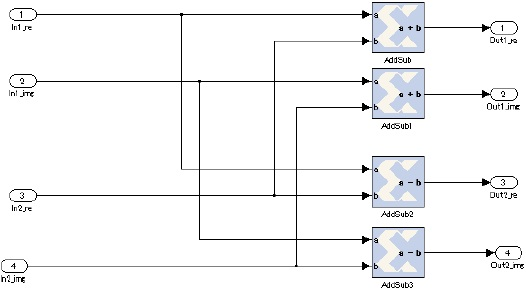Figure 6:  The model of subsystem radix-2_0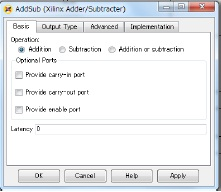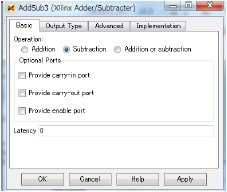b) MULT

This section shows the MULT to the MULT3 subsystem. The MULT subsystem multiplies a twiddle factor. The Complex number operation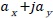mutiple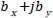is showed by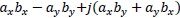As following this process, multiple twiddle factors in terms of complex input. figure 9 shows the circuit block of the MULT subsystem． Abbreviate explanations of the MULT1 subsystem to the MULT3 subsystem, because of the same architecture as the MULT subsystem against the constant block, the W_real and the W_imag of figure 9, parameters.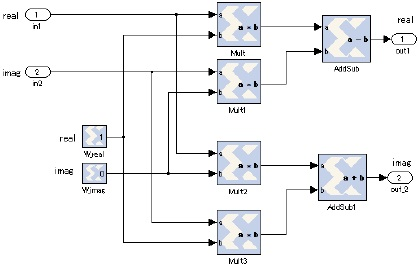Figure 9:  Architecture of the MULT subsystem

Abbreviate an explanation of the AddSub block, because of the same as the AddSub2 block of figure 6.

Also, abbreviate an explanation of the AddSub1 block, because of the same as the AddSub1 block of figure 6.

Figure 10 shows parameters of the Mult block.

Abbreviate explanations of the Mult1 block, the Mult2 block and the Mult3 block, because of the same parameters as the Mult block of figure 9.

Table 1 shows the Constant block parameters showing the twiddle factors depending on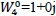or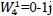in the MULT subsystem, the MULT1 subsystem, the MULT2 subsystem and the MULT3 subsystem.

Table 1:  Parameter of the twiddle factor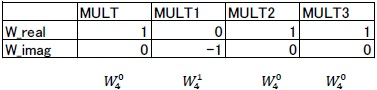The subsystem MULT multiplies the complex twiddle factor in terms of the complex input signals.

### (2) Verification

Input x to x of Table 2 into the FFT circuit of Fig.5, then got X to X of Table 2.

figure 11 shows the result of hardware simulation is consistent with the result of MATLAB simulation.

Table 2:  Verification Result and Error on MATLAB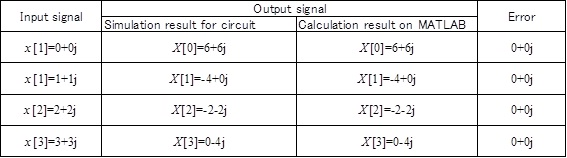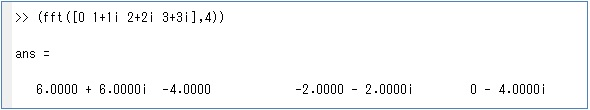Figure 11:  Calculation Result of FFT on MATLAB

Then, figure 12 and figure 13 shows the waveform of input signals and output signals.

Depending on this figure, in case of parallel input, FFT has no latency.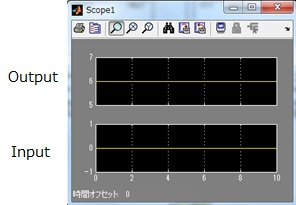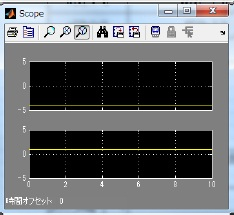Figure 12:  X_real and x_real                Figure 13:  X_real and x_real